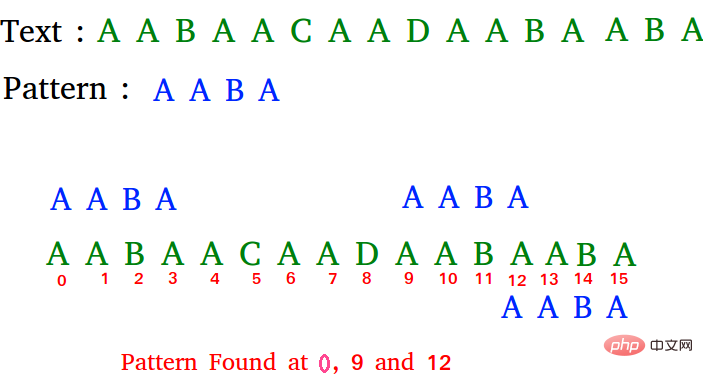# PHP实现用于模式搜索的朴素算法（字符串匹配算法）

```输入:  txt[] = "THIS IS A TEST TEXT"
pat[] = "TEST"

pat[] =  "AABA"

Pattern found at index 9
Pattern found at index 12```PHP代码：

```<?php
// 朴素模式搜索算法

function search(\$pat, \$txt)
{
\$M = strlen(\$pat);
\$N = strlen(\$txt);

for (\$i = 0; \$i <= \$N - \$M; \$i++)
{

// 对于当前索引i，请检查模式匹配
for (\$j = 0; \$j < \$M; \$j++)
if (\$txt[\$i + \$j] != \$pat[\$j])
break;

// if pat[0...M-1] =
// txt[i, i+1, ...i+M-1]
if (\$j == \$M)
echo "Pattern found at index ", \$i."\n";
}
}

\$pat = "AABA";
search(\$pat, \$txt);```

```Pattern found at index 0
Pattern found at index 9
Pattern found at index 13```

```filter_none
brightness_4
pat[] = "FAA";```

1）当文本和图案的所有字符相同时。

```filter_none
brightness_4
txt[] = "AAAAAAAAAAAAAAAAAA";
pat[] = "AAAAA";```

2）当最后一个字符不同时，也会出现最坏情况。

```filter_none
brightness_4
txt[] = "AAAAAAAAAAAAAAAAAB";
pat[] = "AAAAB";```